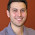Sunday, December 27, 2009

KD-Tree: A C++ and Boost implementation

According to wikipedia a kd-tree (short for k-dimensional tree) is a space-partitioning data structure for organiizing points in a k-dimensional space. In this implementation, points are represented as a boost ublas matrix (numPoints x dimPoints) and the kd-tree can be seen as a row permutation of the matrix. The tree is built recursively. At depth k, the (k % dimPoints) coordinates are analyzed for all the points and the median of them is selected with a quickselect algorithm. The quickselection induces a natural row-index permutation for points in two sets, which are recursively partitioned on the next levels of the tree.

Here you have the code.

1.I can't see the code!!!

2.3.In testKdTree.cpp, the first line is:

KDtree kd (10, 10); // 30 vectors, dim=10

This is not correct. The line would need to be changed to:

KDtree kd (30, 10); // 30 vectors, dim=10

to match the comment.

David